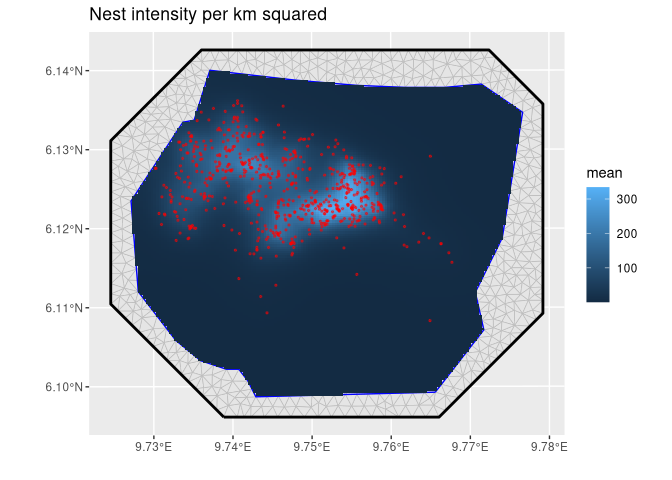The goal of inlabru is to facilitate spatial modeling using integrated nested Laplace approximation via the R-INLA package. Additionally, extends the GAM-like model class to more general nonlinear predictor expressions, and implements a log Gaussian Cox process likelihood for modeling univariate and spatial point processes based on ecological survey data. Model components are specified with general inputs and mapping methods to the latent variables, and the predictors are specified via general R expressions, with separate expressions for each observation likelihood model in multi-likelihood models. A prediction method based on fast Monte Carlo sampling allows posterior prediction of general expressions of the latent variables. See Fabian E. Bachl, Finn Lindgren, David L. Borchers, and Janine B. Illian (2019), inlabru: an R package for Bayesian spatial modelling from ecological survey data, Methods in Ecology and Evolution, British Ecological Society, 10, 760–766, doi:10.1111/2041-210X.13168, and citation("inlabru").

The inlabru.org website has links to old tutorials with code examples for versions up to 2.1.13. For later versions, updated versions of these tutorials, as well as new examples, can be found at https://inlabru-org.github.io/inlabru/articles/

## Installation

You can install the current CRAN version of inlabru:

install.packages("inlabru")

You can install the latest bugfix release of inlabru from GitHub with:

# install.packages("remotes")
remotes::install_github("inlabru-org/inlabru", ref = "stable")

You can install the development version of inlabru from GitHub with

# install.packages("remotes")
remotes::install_github("inlabru-org/inlabru", ref = "devel")

or track the development version builds via inlabru-org.r-universe.dev:

# Enable universe(s) by inlabru-org
options(repos = c(
inlabruorg = "https://inlabru-org.r-universe.dev",
CRAN = "https://cloud.r-project.org"
))

# Install some packages
install.packages("inlabru")

## Example

This is a basic example which shows you how fit a simple spatial Log Gaussian Cox Process (LGCP) and predicts its intensity:

# Load libraries
library(inlabru)
library(INLA)
#> This is INLA_22.12.16 built 2022-12-16 14:25:20 UTC.
#>  - See www.r-inla.org/contact-us for how to get help.
#>  - To enable PARDISO sparse library; see inla.pardiso()
library(ggplot2)

data(gorillas, package = "inlabru")

# Construct latent model components
matern <- inla.spde2.pcmatern(gorillas$mesh, prior.sigma = c(0.1, 0.01), prior.range = c(0.01, 0.01) ) cmp <- coordinates ~ mySmooth(coordinates, model = matern) + Intercept(1) # Fit LGCP model # This particular bru/like combination has a shortcut function lgcp() as well fit <- bru( components = cmp, like( formula = coordinates ~ ., family = "cp", data = gorillas$nests,
samplers = gorillas$boundary, domain = list(coordinates = gorillas$mesh)
),
options = list(control.inla = list(int.strategy = "eb"))
)

# Predict Gorilla nest intensity
lambda <- predict(
fit,
pixels(gorillas$mesh, mask = gorillas$boundary),
~ exp(mySmooth + Intercept)
)

# Plot the result
ggplot() +
gg(lambda) +
gg(gorillas\$nests, color = "red", size = 0.2) +
coord_equal() +
ggtitle("Nest intensity per km squared")